﻿ Effect of Space Charge Rotation with Kapchinskiy-Vladimirskiy Distribution Function in Quadrapole

### Effect of Space Charge Rotation with Kapchinskiy-Vladimirskiy Distribution Function in Quadrapole

N. Morshedian, A. Aminzadeh, N. Abtahi, S. Atashbar TehraniOPEN ACCESSPEER-REVIEWED

## Effect of Space Charge Rotation with Kapchinskiy-Vladimirskiy Distribution Function in Quadrapole

N. Morshedian1,, A. Aminzadeh2, N. Abtahi2, S. Atashbar Tehrani3

1Research School of Plasma Physics and Nuclear Fusion, Tehran-Iran

2Physics Department, Azad Islamic University, Central Tehran Branch, Tehran, Iran

3Physic Department, Azad Islamic Yazd University, Yazd, Iran & School of Particles and Accelerators, Institute for Research in Fundamental Sciences (IPM), ehran, Iran

### Abstract

The effect of space charge rotation has been studied in Vlasov-Poisson potential function with KV distribution in quadrupole. Effective potential varies due to rotation and the related parameter has been obtained based on focusing and defocusing of beam particles. The changed beam plasma frequencies have been calculated in the frame of laboratory coordinates. Also the new Hamiltonian of the system has been derived and finally the equipotential lines, electric and magnetic fields have been obtained. The magnetic field remains without any changing, but the electric field and equipotential lines changed in static qudrupole. The result of rotational space charge in static quadrupole is comparable with rotation of quadrupole system.

### At a glance: Figures

12
Prev Next

• Morshedian, N., et al. "Effect of Space Charge Rotation with Kapchinskiy-Vladimirskiy Distribution Function in Quadrapole." International Journal of Physics 2.2 (2014): 37-40.
• Morshedian, N. , Aminzadeh, A. , Abtahi, N. , & Tehrani, S. A. (2014). Effect of Space Charge Rotation with Kapchinskiy-Vladimirskiy Distribution Function in Quadrapole. International Journal of Physics, 2(2), 37-40.
• Morshedian, N., A. Aminzadeh, N. Abtahi, and S. Atashbar Tehrani. "Effect of Space Charge Rotation with Kapchinskiy-Vladimirskiy Distribution Function in Quadrapole." International Journal of Physics 2, no. 2 (2014): 37-40.

 Import into BibTeX Import into EndNote Import into RefMan Import into RefWorks

### 1. Introduction

In designing circular accelerators for high-intensity beams one has to take into account the combined effect of space charge and lattice imperfections. The issue of envelope resonances and instabilities has received new attention in several papers [1, 2, 3, 4], where as the effect of space charge on linear coupling due to skew drift. In this paper we discuss about Rotation with Kapchinskiy-Vladimirskiy Distribution Function (KV) in [5, 6] and effect on the focusing and defocusing on quadrupole and very important on the designing of accelerators. We introduce the new density of particles in ellipse formed and we find the important effect of this distribution in quadrupole because we have two type of quadrupole that means one of them is focusing system in particles beam and another one is defocusing system in particles beam based on choose the density of particles and drive all formula in Sec.II. and plot the simple accelerators that construct by two focusing and defocusing system quadrupole. In Sec. III we discuses about VLASOV’S EQUATION.

### 2. Formalism

We choose a Kapchinskiy-Vladimirskiy equilibrium distribution function with uniform density profile in configuration space with rotation in ellipse of this distribution.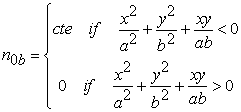(1)

The normal Kapchinskiy-Vladimirskiy equilibrium distribution function with uniform density distribution introduce by: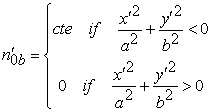(2)

These two type of very effective on the behavior of focusing and defocusing of quadrupole depend on the charge partial. In Eqs. (1-2) a and b the semi axes of the confining ellipse.

Here, it is assumed that the transverse beam dimensions are small in comparison with the radius of the beam pipe so that we can ignore image charges. From Poisson’s equation we readily obtain the effective potential inside the beam valid for a uniform distribution of particles in free space with charge q and line density:(3)

by change the Cartesian space by this equation we have:(4)

In Eq. (4) g denoted by:(5)

The matrix transformation for these rotating is: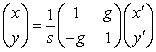(6)

we chose a0, a1 and a2 :(7)

Then we have: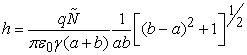(8)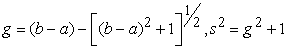(9)

If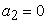this a one quadrupole same as defocusing acting and the Contor Plot for  and Hamiltonian Field Plot also Gradient Field Plot is in fig1 this is a defocusing and if we use the (2) again with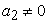we can see this effect in Figure 2 this is a focusing system depend on the charge of particle.

Figure 1. The ContorPlot for Eq.(2) and HamiltonianFieldPlot also GradientFieldPlot show in Figure for defocusing quadrupole with a2 = 0 also a = 500 µm, b = 700 µm and n0;b = 1011 particles

From Poisson’s equation we readily obtain the effective potential inside the beam valid for a uniform distribution of particles in free space with charge q and line density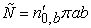(trans-formed to the laboratory frame). After rotating for Eq.(3) we obtain for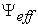effective potential:(10)
Figure 2. The ContorPlot for Eq.(2) and HamiltonianFieldPlot also GradientFieldPlot show in Figure for defocusing quadrupole witha2 = 0 also a = 500 µm, b = 700 µm and n0;b = 1011 particles

By the way we can calculate the Electric field:(11)

and for Kapchinskiy-Vladimirskiy equilibrium distribution function have rotation the Electric field is:(12)
Figure 3. The ContorPlot for Eq.(7) and HamiltonianFieldPlot also GradientFieldPlot show in Figure also also a = 500 µm, b = 700 µm and n0;b = 1011 particles

Eq.(12) plot in Figure 3 and magnetic field in quadrupole is  plot in Figure 4. The magnetic field in the quadrupole is:(13)

in Eq.(13) a distance between two pole and I is current in quadrupole.

Figure 4. The VectorPlot for Eq.(13) and show magnetic field also also n = 1000, I = 50 A and a = 80mm

### 3. Vlasov’S Equation

Withthe beam plasma frequency in the laboratory frame, one can write the actual particle oscillation frequencies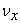,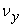in the presence of space charge as: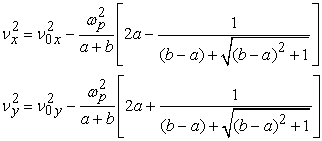(14)

We now assume linear and continuous external focusing forces for the equilibrium beam, and define betatron frequencies in the absence of space charge,corresponding to the oscillation frequencies of a single particle under the applied focusing field in the x and y directions. Considering in addition the self-field effects with their defocusing contribution, we can write the full Hamiltonian as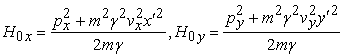(15)

and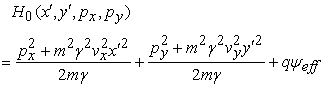(16)

The transverse energy anisotropy T, hence the ratio of oscillation energies in x and y directions, can be written in terms of betatron tunes and emittances as:(17)

The anisotropic KV distribution can now be expressed in terms of a function of the linear combination of the two separate Hamiltonians .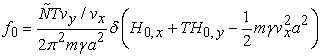(18)

which is consistent with a uniform density with in the boundary of the confining ellipse. We now introduce a lattice error described by a z− component of an error vector potential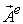. Considering small-amplitude perturbations about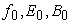and Expressing: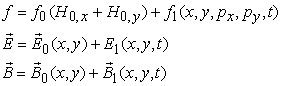(19)

we linearize Vlasov’s equation and keep only first-order terms in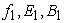to obtain :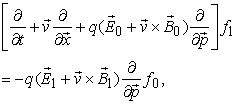(20)

A general remark may be appropriate here. In our approach the equilibrium is a beam matched to the ideal lattice. Turning on errors instantaneously results in time-dependent perturbations of this equilibrium. An alternative approach would be to search for a matched beam in the presence of a gradient or skew error. Plugging into such a system the original matched solution (of the ideal lattice) would then result in oscillations about the error-matched beam another way of looking at the perturbations considered here. With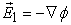and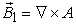we find:(21)

The perturbed electrostatic potential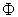in turn self consistently obtained from the linearized Poisson equation: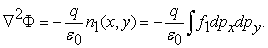(22)

Equations (21) and (22) from a closed set that can be solved with an appropriate boundary

### 4. Result

By rotation we can analyses the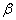function [10, 11, 12, 13]. The origin of the focusing and defocusing are this term x y are describe by Hamiltonian condition also Development of the coupled betatron motion representation intro discuses in refs [14, 15].

### References

  M. Venturini and R. L. Gluckstern, Phys. Rev. ST Accel Beams,3, 034203 (2000).In article CrossRef  A.V.Fedotov and I.Hofmann, Phys. Rev. ST Accel Beams,5, 024202 (2002).In article CrossRef  A.V.Fedotov, I.Hofmann, R.L. Glucksteam, and H.Okamoto, Phys.Rev.ST Accel Beams,6, 094201 (2003).In article CrossRef  M.Aslaninejad, I.Hofmann, Phys. Rev. ST Accel Beams,6, 124202 (2003),J. Padhye, V. Firoiu, D. Towsley, "A stochastic model of TCP Reno congestion avoidance and control", Univ. of Massachusetts, Tech. Rep. 99-02, (1999).In article  H.Qin, M. Chung and R.C.Davidson. Phys. Rev. Lett,103, 224802 (2009).In article CrossRef  H. Qin and R. C. Davidson, Phys. Rev. Lett. 110, no. 6, 064803 (2013).In article CrossRef  Klaus Wille, The Physics of, for supporing him financially for this project Particle Accelerators an introduction.In article  I. Hofmann, Phys. Rev. E,57, 4713 (1998).In article CrossRef  R. C. Davidson and H. Qin, Physics of Intense Charged Particle Beams in High Energy Accelerators (World Scienti_c, Singapore, 2001).In article  V A Lebedev and S A Bogacz, JINST 5 P10010 (2010).In article  R.C. Fernow et al., Possible demonstration of ionization cooling using absorbers in a solenoidal field, in Proceedings of Beam Dynamics and Technology Issues for Muon Collider, Montauk New York U.S.A.,October 1520 1995 AIP Conf. Proc. 372 146 (1996).In article  T.L. Barklow et al., Commissioning experience with the SLC arcs, SLAC-PUB-5056 (1989); T.L. Barklow, P. Emma, P. Krejcik and N.J. Walker, Review of lattice measurement techniques at the SLC, SLAC-PUB-5695 (1991).In article  D. Sagan and D. Rubin, Linear analysis of coupled lattices,Phys. Rev. ST Accel. Beams 2 074001 (1999).In article CrossRef  D.A. Edwards and L.C. Teng, IEEE Trans. Nucl. Sci. 20 885 (1973).In article CrossRef  I. Borchardt, E. Karantzoulis, H. Mais and G. Ripken, Z. Phys. C 39 339 (1988).In article CrossRef